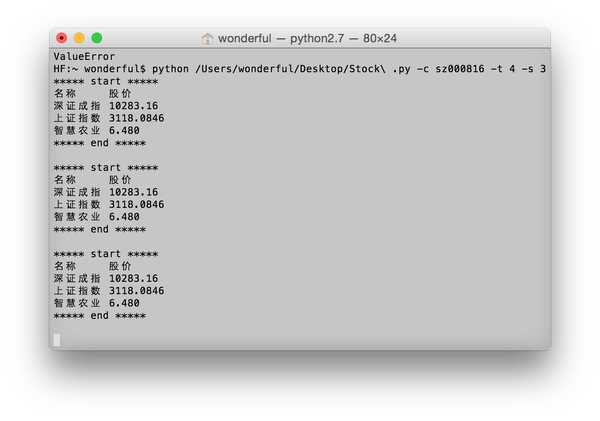Python 实现终端实时获取股票价格

GitHub 里老早之前就 Star 了 felixglow/Stock 这个项目，原作者 felixglow。昨天晚上又想起这个来，于是今儿早上就拿出来看——我对其中相当多的部分都不熟悉，有些还是第一次了解。在这里将我的理解记录下来，其中的错谬之处，还望各位大神指正。

实现效果实现思路

requests请求接口
optparse实现命令行参数处理

具体解析

__author__ = 'felix'
# 原作者为 felix

import requests
# requests 用于爬取新浪股票 API
import time
import sys
# sys 用于在解释器中交互

from Queue import Queue
# Queue 是有关队列的库
from optparse import OptionParser
# OptionParser 用于在命令行中添加选项

# 创建类 Work，多线程获取

def __init__(self, work_queue, result_queue):
# 写入必须绑定的强制属性 self， work_queue， result_queue
# __init__ 方法的第一个参数一定是 self，用于表示创建的实例本身
# 其中，实例是根据类来创建的
# 在 __init__ 方法内部，可将各种属性绑定到 self，因为 self 指向创建的实例本身

self.work_queue = work_queue
self.result_queue = result_queue
self.start()

def run(self):
# 增加一个新方法 run

while True:
func, arg, code_index = self.work_queue.get()
# 获取 func, arg, code_index
res = func(arg, code_index)
self.result_queue.put(res)
if self.result_queue.full():
res = sorted([self.result_queue.get() for i in range(self.result_queue.qsize())], key=lambda s: s, reverse=True)
# sorted() 是用于排序的方法，返回副本，原始输入不变
# Queue.get() 用于获取队列
# Queue.qsize() 返回队列大小
# key=lambda s: s：关键词为 lambda s: s
# lambda s: s：匿名函数，返回第一个元素
# reverse=True：降序排列
res.insert(0, ('0', u'名称     股价'))
# list.insert() 用于将指定对象插入列表的指定位置
print '***** start *****'
for obj in res:
print obj
print '***** end *****\n'

class Stock(object):
# 股票实时价格获取

self.code = code
self.work_queue = Queue()

self.params = self.code.split(',')
# parmas 会向函数传入一个字典
self.params.extend(['s_sh000001', 's_sz399001'])
# 默认获取沪指、深指
# extend()： 扩展，与 append() 的区别为，extend() 加入的元素是分别单个加入的
self.result_queue = Queue(maxsize=len(self.params[::-1]))

self.work_queue.put((self.value_get, stock_code, code_index))
# self.value_get 涉及到下面的装饰器

def del_params(self):
for obj in self.params:

def wait_all_complete(self):
# 判断线程是否是激活的
# 从调用 start() 方法启动线程，到 run() 方法执行完毕或者遇到未处理异常而中断，这段时间内线程是激活的
# join() 的作用是阻塞进程直到线程执行完毕
# 依次检查线程池中的线程是否接触，没有结束就阻塞线程直到线程结束
# 如果结束则跳转执行下一个线程的 join() 函数

@classmethod
# 装饰器，返回函数的高阶函数
def value_get(cls, code, code_index):
slice_num, value_num = 21, 3
name, now = u'——无——', u'  ——无——'
if code in ['s_sh000001', 's_sz399001']:
slice_num = 23
value_num = 1
r = requests.get("http://hq.sinajs.cn/list=%s" % (code,))
# 爬取新浪股票 API
res = r.text.split(',')
if len(res) > 1:
name, now = r.text.split(',')[slice_num:], r.text.split(',')[value_num]
return code_index, name + ' ' + now

if __name__ == '__main__':
# 该脚本用于直接运行，而不能被 import
parser = OptionParser(description="Query the stock's value.", usage="%prog [-c] [-s] [-t]", version="%prog 1.0")
# 生成命令行说明
# %prog 将会以当前程序名的字符串来代替
help="the stock's code that you want to query.")
# dest 是储存的变量
help='How long does it take to check one more time.')
options, args = parser.parse_args(args=sys.argv[1:])
# 设置好命令行后，用 parse_args() 来解析命令行
assert options.codes, "Please enter the stock code!"
# 是否输入股票代码
if filter(lambda s: s[:-6] not in ('sh', 'sz', 's_sh', 's_sz'), options.codes.split(',')):
# 股票代码输入是否正确
raise ValueError

while True:
stock.del_params()
time.sleep(options.sleep_time)
# sleep() 用于使程序休眠